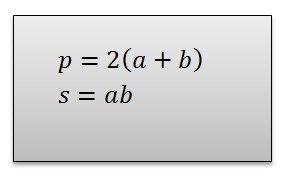Problems

# Perimeter and area

Find the perimeter and the area of rectangle, if we know the lengths of its sides.#### Input

Two integers a and b (a, b109) - the lengths of rectangle sides.

#### Output

Print the perimeter and the area of rectangle.

Time limit 1 second
Memory limit 128 MiB
Input example #1
3 7

Output example #1
20 21

Author Matviychuk Sergiy Volodymyrovych
Source "ABC programming"